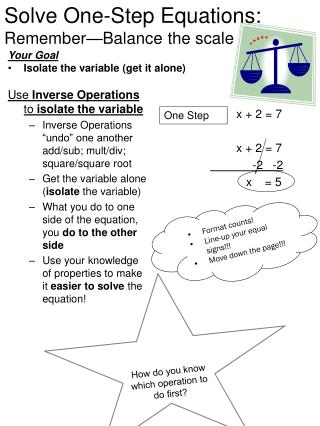# Solve One-Step Equations: Remember—Balance the scale - PowerPoint PPT PresentationDownload PresentationSolve One-Step Equations: Remember—Balance the scale

Solve One-Step Equations: Remember—Balance the scaleDownload Presentation## Solve One-Step Equations: Remember—Balance the scale

- - - - - - - - - - - - - - - - - - - - - - - - - - - E N D - - - - - - - - - - - - - - - - - - - - - - - - - - -
##### Presentation Transcript

1. Your Goal Isolate the variable (get it alone) Solve One-Step Equations:Remember—Balance the scale x + 2 = 7 x + 2 = 7 -2 -2 x = 5 Use Inverse Operations to isolate the variable • Inverse Operations “undo” one another add/sub; mult/div; square/square root • Get the variable alone (isolate the variable) • What you do to one side of the equation, you do to the other side • Use your knowledge of properties to make it easier to solve the equation! One Step • Format counts! • Line-up your equal signs!!! • Move down the page!!! How do youknow which operation to do first?